# New to Qlik Sense

If you’re new to Qlik Sense, start with this Discussion Board and get up-to-speed quickly.

Announcements
Qlik Cloud Maintenance is scheduled between March 27-30. Visit Qlik Cloud Status page for more details.
cancel
Showing results for
Did you mean:Contributor III

## Different totals in the same pivot table

Hello!

I need to do an table in QlikSense like this:There'll be a lot of different products like (Product x, y, z, etc) and I need to see the data by date, and I need to calculate 5 things: sum, max, min, average and standard deviation.

I checked on Qlik that I can add just 1 kind of total: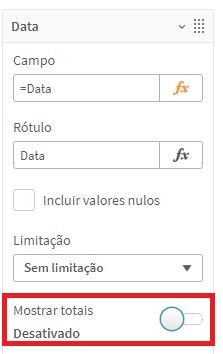How can I add anothers (average, max, min, etc) in this case?

Is there any solution for it?

I'm using a Pivot Table

Labels (1)
• ### dimension

1 Solution

Accepted SolutionsMVP

That's what the set analysis above was trying to do.

You will need to put the same set analysis into all of the total functions, e.g.:

avg({<PivotDim=,Data=P({\$}PivotDim)>}Valor)

You can not do set analysis on an Only function, so you will need to get a bit more creative with that. If you know that there is only ever one value there you can simply do a max:

max({<PivotDim=,Data=P({\$}PivotDim)>}LI)

Hope that helps.

Steve

9 RepliesMVP

I think that you will need to do a bit of hacking to make this work, but it should be possible.

In the load script create a new table that links a PivotDim date to every date in the main table, like this:

PivotDim:
Date,
Date as PivotDim
RESIDENT YourExistingTable;

This will then give you a new dimension you can use in your pivot table, and it will still show the same results for each of the dates.

Now you need to add to that each of the total fields, linking to all the dates so you can aggregate over them. We can use the fact that dates are dual data types (i.e. they have a number and a text value) and create our own dual values, which will sort numerically in the right order.

So, for the sum you would do:

CONCATENATE(PivotDim)
Date,
Dual('SUM', 1) as PivotDim
RESIDENT YourExistingTable;

Now, if you have PivotDim as your dimension and do sum(Value) as the expression it will kind of work, with the values for each date against each date and the total against the SUM row.

Now add in the other totals, being careful to give them each a unique number, in the order you want them:

CONCATENATE(PivotDim)
Date,
Dual('MAX', 2) as PivotDim
RESIDENT YourExistingTable;

etc.

You can also add in the title 'Date' as a new PivotDim.

Once they are all in your sum(Value) expression will give you the total on every one of your aggregation rows.

You now need to adjust the expression based on the PivotDim value, something like this:

if(Only(PivotDim) = 'MAX', max(aggr(sum(Value), Date)),
if(Only(PivotDim) = 'MIN', min(aggr(sum(Value), Date)),
if(Only(PivotDim) = 'AVERAGE', avg(aggr(sum(Value), Date)),
if(Only(PivotDim) = 'Date', '',
sum(Value)))))

If you want a different number format for the Standard Deviation to the rest of the rows you will need to set the number format on the Pivot to Auto and use the num function in the expression and have a similar if statement to populate the number mask correctly.

You will also be able to set the background colour based on the value of the PivotDim, which will allow you to make the totals stand out.

I've not created an example to test this, so you may need to debug things a little, but hopefully that will point you in the right direction.

Cheers,

SteveContributor III
Author

I made the changes as you said, but it didn't work as I expected.

Here is the result: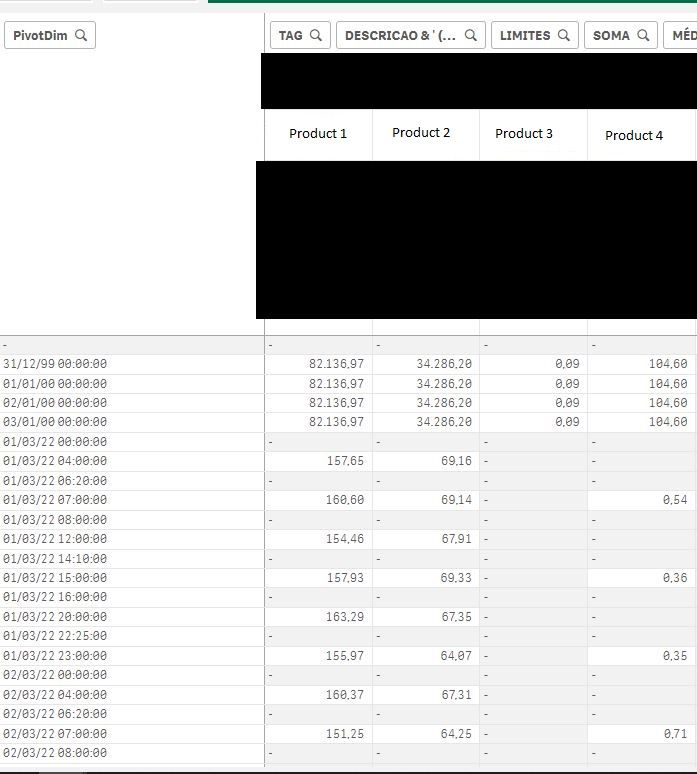And that's my script: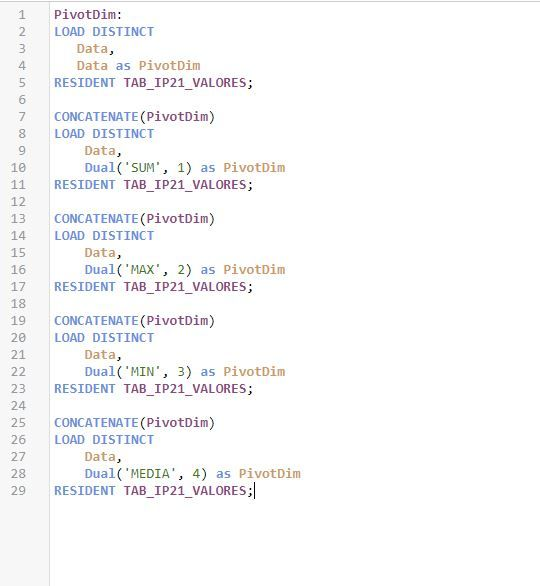MVP

Something you have going on is values against different times, so you want to get rid of the times.

So, in the initial load you would have something like:

Data,
Date(DayStart(Data), 'DD/MM/YYYY') as Day,

Then use Day instead of Data in the PivotDim tables:

PivotDim:
Day,
Day as PivotDim
RESIDENT TAB_IP21_VALORES;

CONCATENATE(PivotDim)
Day,
Dual('MAX', 1) as PivotDim
RESIDENT TAB_IP21_VALORES;

That should get rid of the duplicate rows.

Now you have the issue that the totals are showing as dates. I'm not too sure why that is happening, to be honest.

Can you try with only the totals, so don't load the data island where the individual dates go into PivotDim. You will need to move the table name and the first CONCATENATE to do this. Do the titles then show correctly?

If they do, you could then try adding the dates after all of the other dimensions, rather than at the start - that can make a difference.

Good luck!

SteveContributor III
Author

Hi Steve,

I noticed that when I insert the syntax Dual('SUM','1'), the Qlik transform the number 1 into a date type, in this case: 1/1/00. So, I changed the numbers in my script, because I want to see my data in DESC order: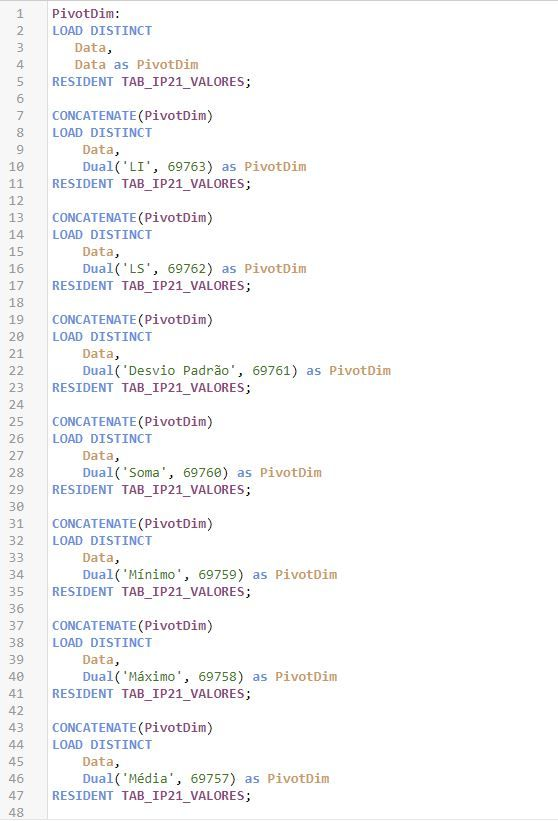After it, I the "Date" dimension, I make this script:

=if(PivotDim = '69763', 'LI',
if(PivotDim = '69762', 'LS',
if(PivotDim = '69760', 'Soma',
if(PivotDim = '69759', 'Mínimo',
if(PivotDim = '69758', 'Máximo',
if(PivotDim = '69757', 'Média', date(PivotDim, 'DD/MM/YY hh:mm:ss'))))))))

So, I got this result:To get the totals (sum, min, max, etc), I make this script:

=if(Only(PivotDim) = '69763', Only(LI),
if(Only(PivotDim) = '69762', Only(LS),
if(Only(PivotDim) = '69761', (StDev(Valor)),
if(Only(PivotDim) = '69760', Sum({<PivotDim>}Valor),
if(Only(PivotDim) = '69759', min(Valor),
if(Only(PivotDim) = '69758', max(Valor),
if(Only(PivotDim) = '69757', avg(Valor), Valor)))))))

The 'last' problem that I have now is that, when I select specific dates, the totals don't work (they disappear), like this:It happens because the field's Soma, Média, Min, Max are dates in the backend, so as I didn't selected them, they don't appear in the selection.

Now I'm trying to figure out a way to fix this field's.MVP

Looks like you have made excellent progress!

I think that to avoid missing data when there are more than one entry in an hour you need to change Valor at the end of the IF block to be sum(Valor).

The thing to do here is to ignore the selection on PivotDim in the selection, but apply the selection to the Data field in Set Analysis.

So, enter the following set analysis into all of the expressions:

sum({<PivotDim=,Data=P({\$}PivotDim)>}Valor)

The first part of the set analysis (PivotDim=) ignores the selection. The next part (Data=P(etc.)) then only selects dates where the date appears in the pivot dim selection. This should fix the situation where dates are selection from the PivotDim field.

It will still break if someone selects one of the totals - but that would be an odd thing to do anyway!

Hope that works for you.

SteveContributor III
Author

Steve,

I added two filters in my sheet: Mês(Month) and Ano(Year).

When I select these filters, the Pivot Table work very well. The fields (Soma, min, max) stay in the top and calculate the totals according to the selection, as here: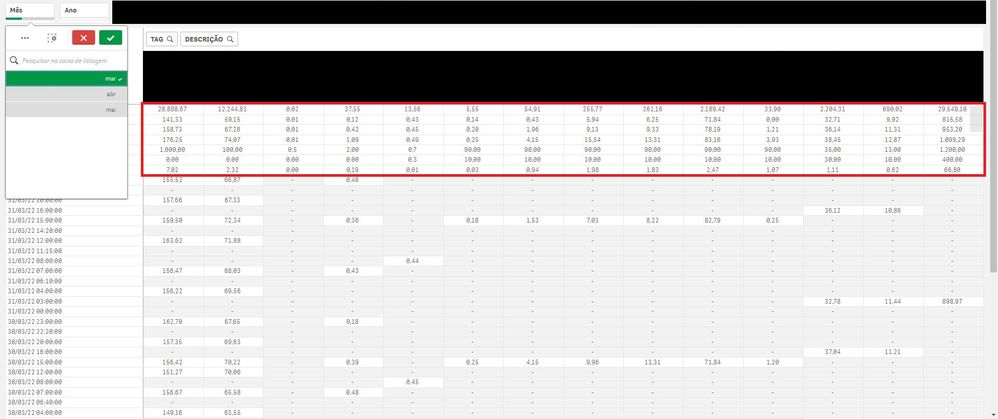But, when I use the filter 'Data' (PivotDim) that is a feature of the PivotTable, the totals disappear, like here: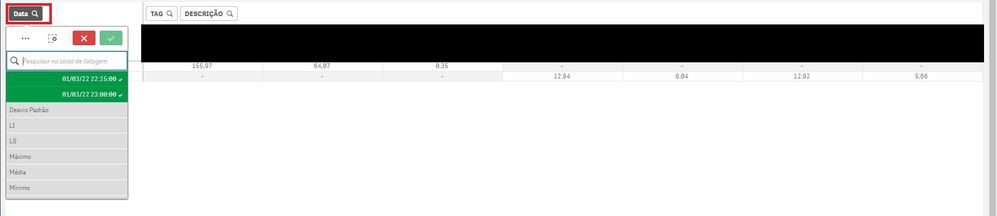So , in this case (when I filter a specific date) I'm trying to figure out a solution to fix (put) the totals (Soma, max, min, etc) in the top and calculate this totals according to my selection.MVP

That's what the set analysis above was trying to do.

You will need to put the same set analysis into all of the total functions, e.g.:

avg({<PivotDim=,Data=P({\$}PivotDim)>}Valor)

You can not do set analysis on an Only function, so you will need to get a bit more creative with that. If you know that there is only ever one value there you can simply do a max:

max({<PivotDim=,Data=P({\$}PivotDim)>}LI)

Hope that helps.

SteveContributor III
Author

Hey, Steve

Thank u so much for the help!MVP

No worries - glad it all worked out.

SteveTags
Community Browser